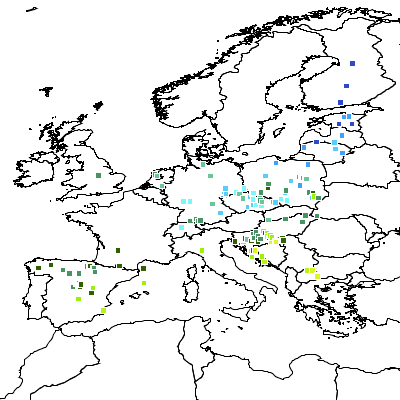## Wednesday 22 February 2012

### A cool application of JTS Voronoi diagrams

I just noticed this series of posts by Stephen Mather on generating medial axes of polygons by creating Voronoi diagrams on the densified linework.

If I read correctly, he's doing this via Python GeoScript, which uses JTS for the heavy lifting.

As he says, this is a venerable technique ( hack) for creating medial axis approximations.  Regardless of its computational soundness (and hey, whatever works), it's a great example of using a series of complex geometric operations to compute a useful result.

I'll have to try this in JEQL....

(Stephen seems to have moved on to implementing the Scale Axis Transform in PGSQL - very impressive!)

## Tuesday 21 February 2012

### Barnes Analysis for Surface interpolation

Recently I've been working on generating surfaces from irregular sets of data observations, for thematic rendering purposes.  The data I'm working with is meteorological measurements (e..g max and min temperature, average rainfall, etc.).  Here's a sample:Maximum Daily Temperatures for November 30, 2010

There are many, many different ways of interpolating surfaces - Wikipedia has a long list.  It turns out that a classic approach for interpolation of meteorological data is Barnes Interpolation (also called Barnes Analysis).  Barnes Interpolation is a surface estimating technique which uses an initial pass to provide a smoothed estimate of the surface, and optionally further refinement passes to improve the surface to more closely match the observation values.  The initial pass produces an averaged (smoothed) surface, using a summation of exponential (Gaussian) decay functions around each observation point.  Subsequent refinement passes compute an error surface using the delta between the previous estimated surface and the observations.  The error surface is added to the previous estimate surface to refine the estimate (by reducing the delta to the observations).

I'm more of a vector guy than a raster guy, but I figured that Google and Wikipedia would make short work of this - after all, how hard can raster be?  Unfortunately, the Wikipedia article is not very helpful - the description is unclear, and it doesn't even define all the symbols used in the presented equations.  I found some better references here and here, but they still contained some confusing discrepancies in the equations.  Barnes' original paper is online as well, but as is often the case with primary sources, it is more concerned with the physical properties of the analysis, and doesn't have a very crisp description of the actual algorithm.

Triangulating between the various sources, I eventually arrived at the following algorithm definition (provided herewith without warranty!):

 Barnes Interpolation Algorithm For the first pass, the estimated value Eg at each grid point gx,y is:    Eg = ∑( wi * oi) / ∑( wi ) where: oi is the value of the i'th observation point wi is the weight (decay function) value for the i'th observation point, defined as       wi = exp(- di2   /   L2C  ) where: di is the distance from the grid point to the i'th observation point L is the length scale, which is determined by the observation spacing and the natural scale of the phenomena being measured.   C is the convergence factor, which controls how much refinement takes place during each refinement step.  In the first pass the convergence factor is 1. For subsequent passes a value in the range 0.2 - 0.3 is effective. During refinement passes the estimate at each grid point is re-computed using:   E'g = Eg + ∑( wi * (oi - Ei) ) / ∑( wi ) where: Ei is the estimated value at the grid cell containing the i'th observation point

The target development platform is Java (of course).  For prototyping purposes I used JEQL to read sample datasets, run the algorithm with various parameter choices, and produce plots to visualize the results.  Here's the surface plot for the dataset above:

This looks pretty reasonable, and provides a nice visualization of the large-scale data trend. (Surprise!  It's hotter down south...)  The ultimate goal is much more exciting.  The algorithm will be provided as a Rendering Transformation in GeoServer, allowing surfaces to be generated dynamically from any supported datastore.  This will open the way to providing all kinds of further surface visualizations, including other interpolation techniques - as well as everyone's favourite, Heat Maps (aka Multivariate Kernel Density Estimation).

Now, the pure mathematical description of the algorithm is sufficient for a proof-of-concept implementation producing single images.  But to make it usable in a dynamic way in a high-demand environment, there are more details which need to be worked out to improve performance and functionality.  For instance:
• How large an extent of data points is necessary to produce a stable surface for a given area?  This is important for dynamic presentation, since zooming, panning and tiling should not change the appearance of the surface.  Of course, all data points could always be used, but more selectivity improves performance.
• How far should the surface be extrapolated into areas of few or no data observations?  Apart from the validity implications, limiting the extent of the computed surface provides a boost in performance.
• A further performance increase may be gained by computing the surface on a coarse grid, then up-sampling in an appropriate way to the display resolution.
• For data which is global in scope, how does the algorithm need to be changed to accommodate computation on the spheroid?  It seems likely that it's necessary to use a geodetic distance calculation, and there may be other impacts as well.